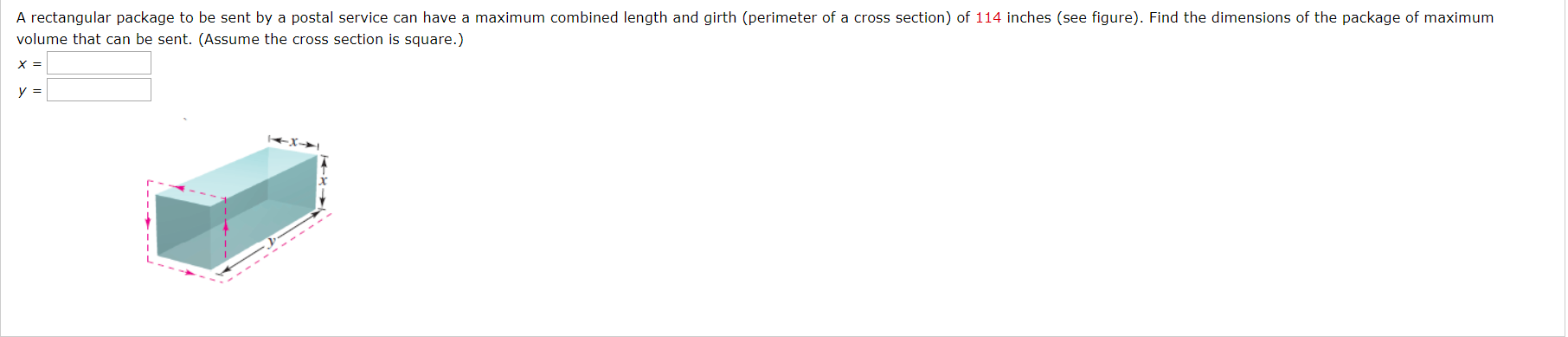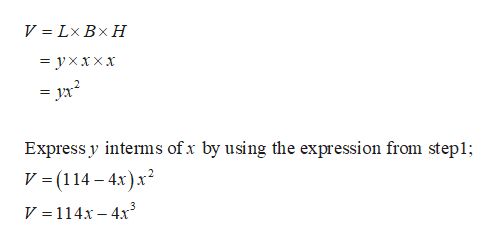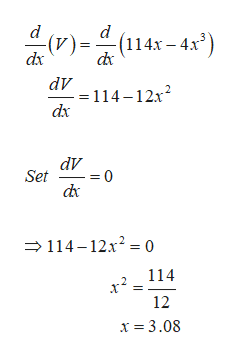# A rectangular package to be sent by a postal service can have a maximum combined length and girth (perimeter of a cross section) of 114 inches (see figure). Find the dimensions of the package of maximumvolume that can be sent. (Assume the cross section is square.)X =y =

Question
44 viewshelp_outlineImage TranscriptioncloseA rectangular package to be sent by a postal service can have a maximum combined length and girth (perimeter of a cross section) of 114 inches (see figure). Find the dimensions of the package of maximum volume that can be sent. (Assume the cross section is square.) X = y = fullscreen
check_circle

Step 1

The combined length and girth can be expressed as,

Step 2

The volume of the rectangular box is given as,help_outlineImage TranscriptioncloseV = Lx Bx H = yxxxx Express y interms of x by using the expression from stepl V (114-4x)x2 V =114x-4x3 fullscreen
Step 3

To maximize the volume, differentiate the Volume functio...help_outlineImage Transcriptionclosed -(114x -4x3) dx dx - (A) dV =114-12x dx dV Set = 0 114-12x2 = 0 114 12 x 3.08 fullscreen

### Want to see the full answer?

See Solution

#### Want to see this answer and more?

Solutions are written by subject experts who are available 24/7. Questions are typically answered within 1 hour.*

See Solution
*Response times may vary by subject and question.
Tagged in
MathCalculus

### Derivative